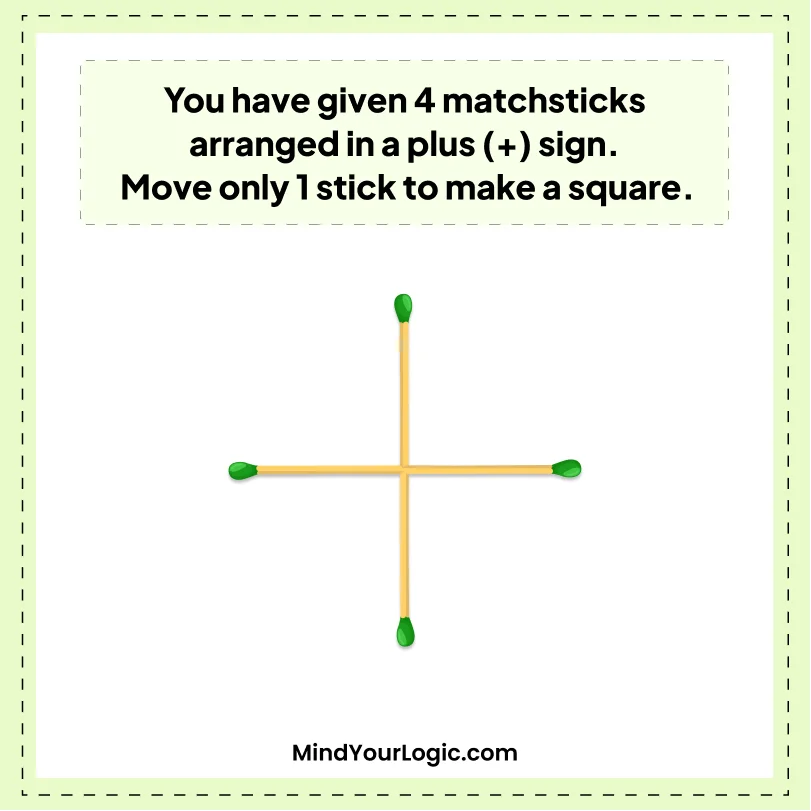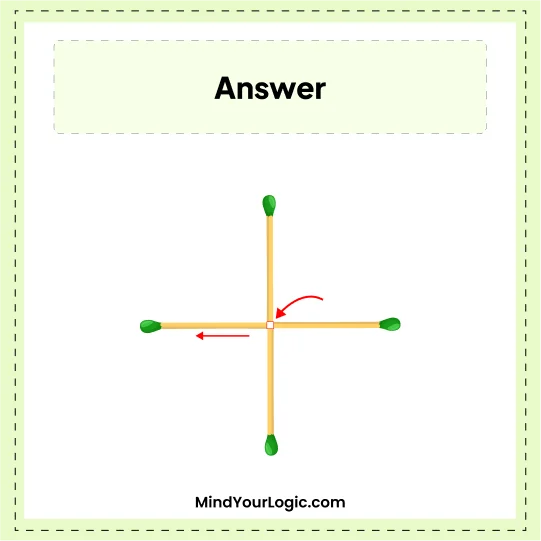# Move 1 matchstick to make Square - Matchstick Puzzle

###### 42.Matchstick Puzzles
`You have given 4 matchsticks arranged in a plus (+) sign. Move only one stick to make a square. `•

Explanation :

``` Arrange 4 extra sticks in Alphabet "Z" and rotate Z then place it inside the square box as given in the below image.
If we cut the square into two parts, we get the square into the same shape and area as two equal parts of the square. ```